# Cryptography: Theory and Practice:Pseudo-random Number Generation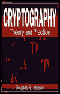Cryptography: Theory and Practice by Douglas Stinson CRC Press, CRC Press LLC ISBN: 0849385210   Pub Date: 03/17/95

## Chapter 12Pseudo-random Number Generation

### 12.1 Introduction and Examples

There are many situations in cryptography where it is important to be able to generate random numbers, bit-strings, etc. For example, cryptographic keys are to be generated at random from a specified keyspace, and many protocols require random numbers to be generated during their execution. Generating random numbers by means of coin tosses or other physical processes is time-consuming and expensive, so in practice it is common to use a pseudo-random bit generator (or PRBG). A PRBG starts with a short random bit-string (a “seed”) and expands it into a much longer “random-looking” bit-string. Thus a PRBG reduces the amount of random bits that are required in an application.

More formally, we have the following definition.

DEFINITION 12.1  Let k,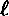be positive integers such that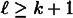(whereis a specified polynomial function of k). A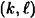- pseudo-random bit generator (more briefly, a-PRBG) is a function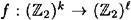that can be computed in polynomial time (as a function of k). The input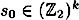is called the seed, and the output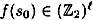is called a pseudo-random bit-string.

The function f is deterministic, so the bit-string f(s0) is dependent only on the seed. Our goal is that the pseudo-random bit-string f(s0) should “look like” truly random bits, given that the seed is chosen at random. Giving a precise definition is quite difficult, but we will try to give an intuitive description of the concept later in this chapter.

One motivating example for studying this type PRBG is as follows. Recall the concept of perfect secrecy that we studied in Chapter 2. One realization of perfect secrecy is the One-time Pad, where the plaintext and the key are both bitstrings of a specified length, and the ciphertext is constructed by taking the bitwise exclusive-or of the plaintext and the key. The practical difficulty of the One-time Pad is that the key, which must be randomly generated and communicated over a secure channel, must be as long as the plaintext in order to ensure perfect secrecy. PRBGs provide a possible way of alleviating this problem. Suppose Alice and Bob agree on a PRBG and communicate a seed over the secure channel. Alice and Bob can then both compute the same string of pseudo-random bits, which will be used as a One-time Pad. Thus the seed functions as a key, and the PBRG can be thought of as a keystream generator for a stream cipher.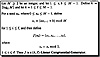Figure 12.1  Linear Congruential Generator

We now present some well-known PRBGs to motivate and illustrate some of the concepts we will be studying. First, we observe that a linear feedback shift register, as described in Section 1.1.7, can be thought of as a PRBG. Given a k-bit seed, an LFSR of degree k can be used to produce as many as 2k - k - 1 further bits before repeating. The PRBG obtained from an LFSR is very insecure: we already observed in Section 1.2.5 that knowledge of any 2k consecutive bits suffice to allow the seed to be determined, and hence the entire sequence can be reconstructed by an opponent. (Although we have not yet defined security of a PRBG, it should be clear that the existence of an attack of this type means that the generator is insecure!)

Another well-known (but insecure) PRBG, called the Linear Congruential Generator, is presented in Figure 12.1. Here is a very small example to illustrate.

Example 12.1

We can obtain a (5, 10)-PRBG by taking M = 31, a = 3 and b = 5 in the Linear Congruential Generator. If we consider the mapping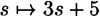mod 31, then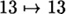, and the other 30 residues are permuted in a cycle of length 30, namely 0, 5, 20, 3, 14, 16, 22, 9, 1, 8, 29, 30, 2, 11, 7, 26, 21, 6, 23, 12, 10, 4, 17, 25, 18, 28, 27, 24, 15, 19. If the seed is anything other than 13, then the seed specifies a starting point in this cycle, and the next 10 elements, reduced modulo 2, form the pseudo-random sequence.

Table 12.1 Bit-strings produced by the linear congruential generator
seed   sequence
0 1010001101
1 0100110101
2 1101010001
3 0001101001
4 1100101101
5 0100011010
6 1000110010
7 0101000110
8 1001101010
9 1010011010
10 0110010110
11 1010100011
12 0011001011
13 1111111111
14 0011010011
15 1010100011
16 0110100110
17 1001011010
18 0101101010
19 0101000110
20 1000110100
21 0100011001
22 1101001101
23 0001100101
24 1101010001
25 0010110101
26 1010001100
27 0110101000
28 1011010100
29 0011010100
30 0110101000

The 31 possible pseudo-random bit-strings produced by this generator are illustrated in Table 12.1.

We can use some concepts developed in earlier chapters to consrtruct PRBGs. For example, the output feedback mode of DES, as described in Section 3.4.1, can be thought of as a PRBG; moreover, it appears to be computationally secure.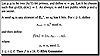Figure 12.2  RSA Generator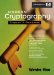Modern Cryptography: Theory and Practice
ISBN: 0130669431
EAN: 2147483647
Year: 1995
Pages: 133
Authors: Wenbo Mao

Similar book on Amazon